# RBSE Maths Class 11 Chapter 9: Important Questions and Solutions

RBSE Solutions For Class 11 Maths Chapter 9 – Sequence, progression and series available here. The important questions and solutions of Chapter 9, available at BYJU’S, contain step by step explanations for the better understanding of the students. All these important questions are based on the new pattern prescribed by the RBSE. Students can also get the syllabus and textbooks on RBSE Class 11 solutions.

Chapter 9 of the RBSE Class 11 Maths will help the students to solve problems related to the sequence, series, progression, arithmetic progression, sum to n terms of an arithmetic progression, arithmetic mean, properties of arithmetic progression, geometric progression, geometric mean, the relation between arithmetic and geometric mean, sum to n terms of a geometric progression, the sum of an infinite GP, arithmetic and geometric series, sum to n terms of a series, harmonic progression, harmonic mean, relation between the means. After referring to these important questions and solutions, you can refer to 2020-2021 solutions for the chapter here.

### RBSE Maths Chapter 9: Exercise 9.1 Textbook Important Questions and Solutions

Question 1: From the following sequences which are in A.P.?

(i) 2, 6, 11, 17,…

(ii) 1, 1.4, 1.8, 2.2,…

(iii) -7, -5, -3, -1,..

(iv) 1, 8, 27, 64,…

Solution :

Given sequence will be in A.P. only if the common difference of two consecutive terms is the same.

 Serial number Given sequence Difference between the first and second term Difference between the second and third term Is the given sequence an AP or not? (i) 2, 6, 11, 17,… 6 – 2 = 4 11 – 6 = 5 No (ii) 1, 1.4, 1.8, 2.2,… 1.4 – 1 = 0.4 1.8 – 1.4 = 0.4 Yes (iii) -7, -5, -3, -1,.. (-5) – (-7) = 2 (-3) – (-5) = 2 Yes (iv) 1, 8, 27, 64,… 8 – 1 = 7 27 – 8 = 19 No

Question 2: Find the first term, common difference and the 5th term of given sequences which have the following nth terms:

(i) 3n+ 7

(ii) a + (n – 1) d

(iii) 5 – 3n

Solution:

(i) 3n + 7

nth term, Tn = 3n + 7

First term T1 = 3 × 1 + 7 = 3 + 7 = 10

Second term T2 = 3 × 2 + 7 = 6 + 7 = 13

Common difference

d = T2 – T1

= 13 – 10

= 3

Fifth term T5 = 3 × 5 + 7 = 15 + 7 = 22

Hence, a = 10, d = 3 and T5 = 22

(ii) a + (n – 1) d

nth term Tn = a + (n – 1) d

First term T1 = a + (1 – 1) d

= a + 0 × d

= a + 0

= a

Second term T2 = a + (2 – 1) d

= a + d

Common difference

d = T2 – T1

= a + d – a

= d

Fifth term T5 = a + (5 – 1) d

= a + 4d

Hence, T5 = a + 4d

(iii) 5 – 3n

nth term Tn = 5 – 3n

First term,

T1 = 5 – 3 × 1

= 5 – 3

= 2

Second term

T2 = 5 – 3 × 2

= 5- 6

= -1

Common difference

d = T2 – T1

= (- 1) – 2

= -3

Fifth term

T5 = 5 – 3 × 5

= 5 – 15

= – 10

Hence, a = 2, d = -3 and T5 = – 10.

Question 3: Show that the following sequences with their nth terms are not in arithmetic progression.

[i] [n] / [n + 1]

[ii] n2 + 1

Solution:

If the value of Tn +1 – Tn is not independent of n then the sequence of nth terms are not in arithmetic progression.

[i] [n] / [n + 1]

Tn +1 – Tn = {[n + 1] / [n + 1] + 1} – {[n] / [n + 1]}

= {[n + 1] / [n + 2]} – {[n] / [n + 1]}

= {[n + 1]2 – [n(n +2)]} / [(n + 1) (n + 2)]

= {(n2 + 2n + 1 – n2 – 2n)} / [(n + 1) (n + 2)]

= 1 / [(n + 1) (n + 2)]

Hence, the given sequence with its nth term is not in arithmetic progression.

[ii] n2 + 1

Tn +1 – Tn = {(n+ 1)2 + 1} – {n2 + 1}

= (n2 + 1 + 2n + 1) – (n2 + 1)

= (n2 + 2n + 2 – n2 – 1)

= 2n + 1

Hence, the given sequence with its nth term is not in arithmetic progression.

Question 4: In an A.P. 2 + 5 + 8 + 11 +… which term is 65?

Solution:

2 + 5 + 8 + 11 + ….

Let the nth term be 65.

Then Tn = 65 and d = 5 – 2 = 3

Tn = a + (n – 1) d

⇒ a + (n – 1) d = 65

⇒ 2 + (n – 1) × 3 = 65

⇒ 2 + 3n – 3 = 65

⇒ 3n – 1 = 65

⇒ 3n = 65 + 1

⇒ 3n = 66

⇒ n = 66 / 3

⇒ n = 22

Hence, the 22nd term is 65.

Question 5: In an A.P. 4 + 9 + 14 + 19 +… + 124, find the 13th term from the last.

Solution:

4 + 9 + 14 + 19 +…+ 124

a = 4, d = 9 – 4 = 5, L = 124

13th term from last

= L – (n – 1) d

= 124 – (13 – 1) × 5

= 124 – 12 × 5

= 124 – 60

= 64

Hence, the 13th term from the last is 64.

Question 6: In an A.P. 2 + 5 + 8 + 11 + … if the last term is 95, then find the number of terms of the series.

Solution:

2 + 5 + 8 + 11 + … + 95

a = 2, d = 5 – 2 = 3, l = 95

Then, from l = a + (n – 1 ) d

⇒ 2 + (n – 1) × 3 = 95

⇒ 3 (n – 1) = 95 – 2

⇒ (n – 1) = 93 / 3

⇒ (n – 1) = 31

⇒ n = 31 + 1

= 32

Hence, the number of terms of the series is 32.

Question 7: If the 9th term of A.P. is zero, then prove that 29th term is twice the 19th term.

Solution:

Let a be the first term and d be the common difference then 9th term = T9 = 0

Then a + (9 – 1) d= 0

⇒ a + 8d = 0 ….. (i)

29th term = T29 = a + 28d … (ii)

19th term = T19 = a + 18d … (iii)

Putting the value of equation (i) into equation (ii) and (iii)

T29 = (a + 8d) + 20d

⇒ T29 = 0 + 20 d

⇒ T29 = 20d … (iv)

⇒ T19= (a + 8 d) + 10 d

⇒ T19 = 0 + 10d

⇒ T19 = 10d … (v)

From equation (iv) and (v), we have

T29 = 20d = 2 × 10d = 2 × T19

T29 = 2 T19

Question 8: How many two-digit natural numbers are divisible by 3?

Solution:

The first two-digit number which is divisible by 3 is 12 and the last two-digit number is 99.

To find the numbers which are divisible by 3, the common difference is 3.

a = 12, d = 3, l = 99

Hence, l = a + (n – 1 )d

⇒ 12 + (n – 1) × 3 = 99

⇒ 3 (n – 1) = 99 – 12

⇒ n = 29 + 1

= 30

Hence, the two digit natural numbers which are divisible by 3 are 30.

Question 9: If in A.P., pth term is q and qth term is p, then find (p + q)th term.

Solution:

Let the first term of A.P. = a and common difference = d

Then pth term = Tp = a + (p – 1) d = q

⇒ a + (p – 1) d = q … (i) and

qth term = Tp = a + (q – 1) d = p

⇒ a + (q – 1)d = p …. (ii)

(pth + qth term) = Tp+q = a+ {(p + q) -1} …. (iii)

Subtracting equation (ii) from (i),

{a + (p – 1)d} – {a + (q – 1)d} = q – p

⇒ a + (p – 1) d – a – (q – 1)d = q – p

⇒ (p – 1 – q + 1 )d = q – p

⇒ (p – q)d – (p – q)

⇒ d = -1

Putting value of d in equation (i)

a + (p- 1) (- 1) = q

⇒ a – (p – 1) = q

⇒ a = q + p – 1

⇒ a = p + q – 1

Putting the value of a and d in equation (iii),

Tp+q= a + [(p + q) -]d

= (p + q – 1) + [(p + q -1)(- 1)]

= (p + q -1) – (p + q – 1)

= 0

Hence, (p + q)th term is zero.

Question 10: In an A.P. pth term is 1/q and 17th term 1/p, then prove that pqth term is unity.

Solution:

Let the first term be a and common difference be d.

pth term = Tp = a + (p – 1) d = 1 / q

a + (p – 1) d = 1 / q —- (1)

qth term = Tq = a + (q – 1) d = 1 / p

a + (q – 1) d = 1 / p —- (2)

Solving equations (1) and (2),

d = 1 / pq and a = 1 / pq

pqth term Tpq = a + (pq – 1) d —- (3)

Subtract equation (2) from (1),

a + (p – 1) d = 1 / q —- (1)

a + (q – 1) d = 1 / p —- (2)

(pd – d) – (qd – d) = [1 / q] – [1 / p]

(p – q)d = [p – q] / pq

d = 1 / pq

Putting the value of d in equation (1),

a + (p – 1) * (1 / pq) = 1 / q

a + (1 / q) – (1 / pq) = 1 / q

a = 1 / pq

Putting the values of a and d in equation (3),

apq = a + (pq – 1) d

apq = [1 / pq] + (pq – 1) * (1 / pq)

apq = 1

### RBSE Maths Chapter 9: Exercise 9.2 Textbook Important Questions and Solutions

Question 1: Find the sum of the following progression 7 + 11 + 15 + 19 + …… upto 20 terms.

Solution:

7 + 11 + 15 + 19 + …

Here, a = 7, d = 11 – 7 = 4, n = 20

Then Sn = [n / 2] * [2a + (n – 1)d]

S20 = [20 / 2] * [2 * 7 + (20 – 1) * 4]

= 10 * [14 + 19 × 4]

= 10 × (14 + 76)

= 10 × 90

= 900

Hence, the sum of 20 terms is 900.

Question 2: Find the sum of the odd integers from 1 to 101, which is divisible by 3.

Solution:

Odd integers from 1 to 101 are 1, 3, 5, 7, 9, …, 101

Integers which are divisible by 3 are 3, 9, 15, 21, …… 99.

First term of this series a = 3, common difference d = 9 – 3 = 6, last term = 99 and let the number at the last term be n, then the last term

= 3 + (n – 1) × 6 = 99 [From formula, l = a + (n + 1 )d]

⇒ 6 (n – 1) = 99 – 3

⇒ (n – 1) = 96 / 6

⇒ n = 16 + 1

= 17

Hence, the sum of 17 terms

Sn = [n / 2] * [2a + (n – 1)d]

S17 = [17 / 2] * [2 * 3 + (17 – 1) * (6)]

= [8.5] * [6 + 96]

= 8.5 * 102

= 867

Hence, the sum of odd integers from 1 to 101, which is divisible by 3 is 867.

Question 3: Find the sum of n terms of A.P. whose rth term is 2r + 3.

Solution:

Tr = 2r + 3

Then, Tn = (2n + 3)

Hence, T1 = 2 × 1 + 3

= 2 + 3

= 5 and

T2 = 2 × 2 + 3

= 4 + 3

= 7

Common difference

d = T2 – T1

= 7 – 5

= 2

∴ Sum of n terms, Sn = [n / 2] * [2a + (n – 1)d]

= [n / 2] * [2 * 5 + (n – 1) * 2]

= [n / 2] * [10 + 2n – 2]

= [n / 2] * [8 + 2n]

= n [4 + n]

Hence, the sum of n terms is n (n + 4).

Question 4: If the sum of n terms of A.P. 1, 6, 11,…. is 148, then find the number of terms and last term.

Solution:

Given progression = 1, 6, 11, …

Sn = 148, a = 1,d = 6 – 1 = 5

∵ Sn = [n / 2] * [2a + (n – 1)d]

⇒ [n / 2] * [2 × 1 + (n – 1) × 5] = 148

⇒ [n / 2] * [2 + 5n – 5] = 148

⇒ n (5n – 3) = 296

⇒ 5n2 – 3n – 296 = 0

On solving,

⇒ 5n² – 40n + 37n – 296 = 0

⇒ 5n( n – 8) + 37( n – 8) = 0

⇒ (n – 8) (5n + 37) = 0

⇒ n – 8 = 0 or 5n + 37 = 0

⇒ n = 8 or n = -37 / 5

Since the number of terms cannot be fraction or a negative number, n = 8.

Therefore n is the 8th term.

Tn = T8 = a + (n – 1) d

= 1 + (8 – 1) 5

= 1 + 7 (5)

= 1 + 35

= 36

8th term = n = 36.

Question 5: If the sum of n, 2n, 3n terms of any A.P. are S1, S2 and S3 respectively, then prove that S3 = 3 (S2 – S1).

Solution:

Let a be the first term and d be the common difference of an A.P. then,

Sum to n terms = S1 = [n / 2] * [2a + (n – 1)d] —– (1)

Sum to 2n terms = S2 = [2n / 2] * [2a + (2n – 1)d] —- (2)

Sum to 3n terms = S3 = [3n / 2] * [2a + (3n – 1)d] —- (3)

Subtracting equation (1) from equation (3),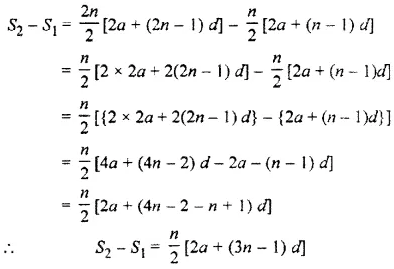Multiplying by 3 on both sides,

3 (S2 – S1) = [3n / 2] [2a + (3n – 1) d]

= S3 [from equation (3)]

Therefore, S3 = 3 (S2 – S1).

Question 6: Find the sum of A.P. a1 + a2 + a3…, a30, given that a1 + a7 + a10 + a21 + a24 + a30 = 540.

Solution:

The number of terms in series = 30

Tr +Tr – 1 = a + 1

∵ a7 is 7th term from starting and a24 is 7th term from the end,

a7 + a24 = a1+ a30 …(i)

Similarly, a10 is 10th term from starting and a21 is 10th term from the end, then

a10 + a21 = a1+ a30

a1 + a7 + a10 + a21 + a24 + a30 = 540

⇒ (a1 + a30) + (a7 + a24) + (a10+ a21) = 540

From equation (i) and (ii),

(a1 + a30) + (a1 + a30) + (a1+ a30) = 540

⇒ (a1 + a30) = 540

⇒ (a1 + a30) = 180

Hence, sum of 30 terms,

S30 = (a1 + a30)

= 15 (180)

= 2700

Hence, a1 + a2 + a3…, a30 = 2700.

Question 7: The interior angles of a polygon are in A.P. The smallest interior angle is 52° and if the difference of consecutive interior angles is 8°, then find the number of sides of the polygon.

Solution:

Smallest angle = 52°

The difference between consecutive angles = 8°

Let the number of sides of a polygon be x.

First-term a = 52°

Common difference, d = 8°

Sum of interior angles = (n – 2) * 360°

S1 = [n / 2] * [2a + (n – 1)d]

⇒ (n – 2) 360° = [n / 2] * [2 x 52° + (n – 1) 8°]

⇒ 360°n – 720° = 104°n + 8°n2 – 8°n

⇒ 8°n2 + (104° – 360° – 8°)n + 720° = 0

⇒ 8°n2 – 264°n + 720° = 0

⇒ n2 – 33°n + 90° = 0

On solving, n = 3 or 30

But n ≠ 30 because, for n = 30, we get

Last angle dn = a + (n + 1)d

= 52° + (30 – 1)8°

= 52° + 29 × 8° = 52° + 232°

= 284° which is impossible

Hence, the number of sides is 3.

### RBSE Maths Chapter 9: Exercise 9.3 Textbook Important Questions and Solutions

Question 1: Find the 7th term of the series 1 + 3 + 9 + 27 + ……..

Solution:

Given series = 1 + 3 + 9 + 27 + ….

Here, a = 1, r = 3 / 1 = 3

7th term = T1 (From Tn = arn-1)

= 1 × (3)7-1

= (3)6

= 729

Question 2: Which term of series 64 + 32 + 16 + 8 + … is 1 / 64?

Solution:

64 + 32 + 16 + 8 + …

Let the nth term be 1 / 64.

a = 64, r = 32 / 64 = [1 / 2]

Tn = arn-1

[1 / 64] = 64 * [(1 / 2)]n-1

[(1 / 2)]n-1 = 1 / [64 * 64] [(1 / 2)]n-1 = 1 / 4096

[(1 / 2)]n-1 = [1 / 2]12

On comparing the powers, since the bases are the same, we get,

n – 1 = 12

n = 12 + 1

n = 13

The 13th term is [1 / 64].

Question 3: The third term of a G.P. is 32 and 7th term is 8192, then find 10th term of GP.

Solution:

T3 = ar2 = 32 —- (1)

T7 = ar6 = 8192 —– (2)

Divide equation (ii) by (i), we get

⇒ r4 = 256

⇒ r4 = 44

On comparing r = 4

Then, from equation (i)

ar2 = 32

⇒ a × 42 = 32

⇒ a = 2

10th term

= T10

= ar9

= 2 × (4)9

= 524288

Question 4: Find the three G.M. between 3 and 48.

Solution:

Let G1, G2, G3 be the three GM between 3 and 48, then

3, G1, G2, G3, 48 will be in G.P.

a = 3, last term (5th term) = 48 and n = 5

3 * r4 = 48 [∵ l = arn – 1 ]

⇒ r4 = 16 = 24

⇒ r = 2

G1 = 3 × 2 = 6

G2 = 3 × 22 = 12

G3 = 3 × 23 = 24

Hence, the three G.M. are 6, 12, 24.

Question 5: If 4th term of any G.P. is p, 7th term is q and 10th term is r, then prove that q2 – pr.

Solution:

Let a is the first term and r is the common ratio of G.P., then according to the question

T4 = ar4 – 1

p = ar3 ….. (i)

T7 = ar7 – 1

q = ar6 ….. (ii)

T10 = ar10 – 1

r = ar9 ….. (iii)

Multiplying equation (i) and (iii), we get

a2 r12 = pr ….. (iv)

Squaring of equation (ii),

q2 = a2r12

Hence, from equation (iv) and (v)

q2 = pr

Question 6: If a, b, c are in G.P. and ax = by = cz, then prove that [1 / x] + [1 / z] = [2 / y].

Solution:

ax = by = cz = k

Then, a = k1/x

b = k1/y

c = k1/z and a, b and c are in GP.

b2 = ac —- (1)

Putting the value of a, b and c in equation (1),

[k1/y]2 = k1/x * k1/z

k2/y = k1/x+1/z is possible when [1 / x] + [1 / z] = [2 / y].

Question 7: If n G.M. inserted between a and b then prove that the product of all geometric means will be (√xy)n.

Solution:

Let G1, G2, G3, G4, ……Gn are in G.M. in between x and y then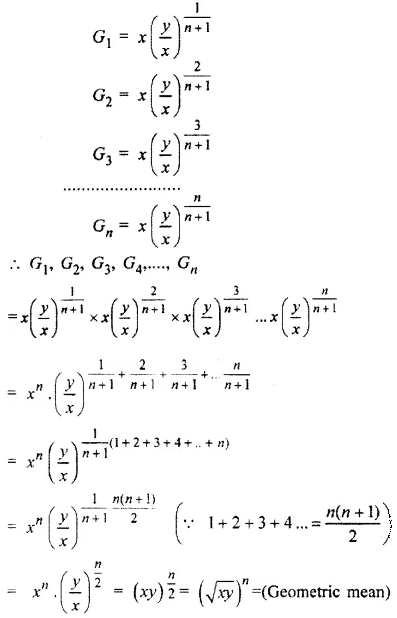### RBSE Maths Chapter 9: Exercise 9.4 Textbook Important Questions and Solutions

Question 1: Find the sum of the geometric progression 2 + 6 + 18 + 51 + …….. upto 7 terms.

Solution:

2 + 6 + 18 + 51 + …….. upto 7 terms

a = 2, r = 6 / 2 = 3, n = 7 {r > 1}

Sn = a[rn – 1] / [r – 1]

S7 = 2[37 – 1] / [3 – 1]

S7 = 2[37 – 1] / 2

S7 = [37 – 1]

S7 = 2187 – 1

= 2186

Question 2: Find the sum of the geometric progression 2 + 6 + 18 + 54 + … + 486.

Solution:

2 + 6 + 18 + 54….+ 486

a = 2, r = 6 / 2 = 3, Tn = 486 (r > 1)

nth term Tn = arn – 1

arn – 1 = 486

2 * (3)n – 1 = 486

⇒ (3)n – 1 = 243 = (3)5

⇒ n – 1 = 5

n = 5 + 1 = 6

Sum of n terms

Sn = a[rn – 1] / [r – 1]

S6 = 2 [36 – 1] / [3 – 1]

S6 = 36 – 1

= 729 – 1

= 728

Hence, the sum is 728.

Question 3: Find the sum of first n terms of the following series 7 + 11 + 777 + …

Solution:

7+ 77 + 777 + 7777 upto n terms.

It is clear that this series is not in G.P. but it can be related to G.P. by writing it in the following form.

Sn = 7 + 77 + 777 + 7777 + … upto n terms

= 7[1 + 11 + 111 + 1111 + … upto n terms]

= [7 / 9] [9 + 99 + 999 + …. upto n terms]

= [7 / 9] [(10 – 1) + (102 – 1) + (103 – 1) …… upto n terms]

= {70[10n – 1] / 81} – [7n / 9]

Question 4: Find the rational form of the recurring decimal 2.3555555.

Solution:

Digit 5 is repeated in 2.35.

∴ 2.35 = 2.35555…………

= 2 + [.3 + .05 + .005 + .0005+ … upto infinity]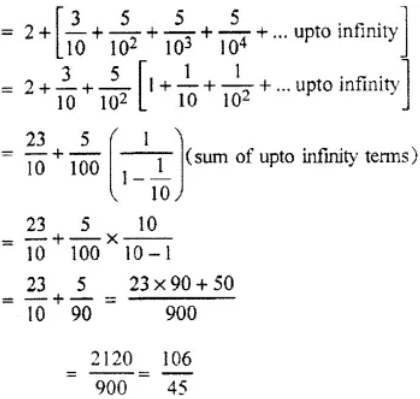Question 5: If y = x + x2 + x3 + … ∞, where | x | < 1, then prove that x = [y] / [1 + y].

Solution:

y = x + x2 + x3 + … ∞

⇒ y = x ( 1 + x + x2 + … ∞)

⇒ y = x (1 / [1 – x])

⇒ y (1 – x) = x

⇒ y – xy – x =0

⇒ y – x(y + 1) =0

⇒ x(1 + y) = y

⇒ x = [y] / [1 + y]

### RBSE Maths Chapter 9: Exercise 9.5 Textbook Important Questions and Solutions

Question 1: Find the sum of n terms of the series 1 + 1 + [3 / 22] + [4 / 23] + ……

Solution:

Let the sum of the series be Sn.

Sn = 1 + [2 / 2] + [3 / 22] + [4 / 23] + ……

In this, 1, 2, 3, 4 ….. is AP and 1, [1 / 2], [1 / 22], ….. is a GP.

nth term of an AP = 1 + (n – 1)n = n

nth term of a GP = 1 * (1 / 2)n-1 = 1 / 2n-1

The arithmetic-geometric series is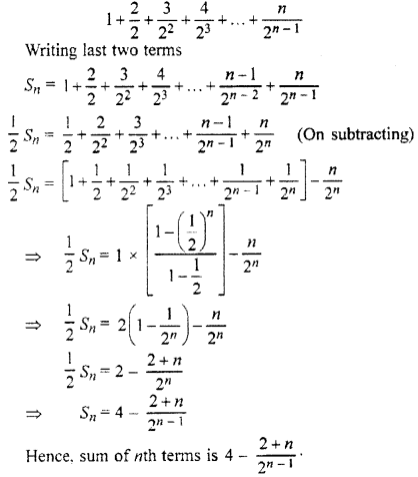Question 2: Find the sum of infinite terms of the series [3 / 7] + [5 / 21] + [7 / 63] + [9 / 189] ……

Solution:

Let the sum of series be S.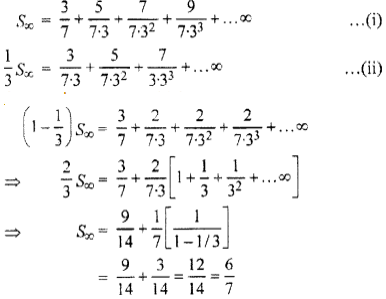The sum of the series is [6 / 7].

Question 3: Find nth term and the sum of n terms of the series 3.2 + 5.22 + 7.23 + …

Solution:

In the given series, A.P. is 3, 5, 7, …

The nth term = 3 + (n – 1) 2

= 3 + 2n – 2

= 2n + 1

The G.P. is 2, 22 , 23 , … and its nth term is 2n.

Let the sum of its n terms be Sn and nth term be Tn.

The nth term of given arithmetic-geometric series is Tn = (2n + 1)2n.

Let the sum of series be Sn on putting the value of n in this, we get

Sn = 3.2 + 5.22 + 7.23 + … + … + (2n – 1)2n + 1 + (2n+ 1)2n …(i)

2Sn = 3.22 + 5.22 + 7.23 …+ (2n – 1)2n + (2n + 1)2n + 1 …(ii)

Subtracting equation (ii) from (i), we get

-Sn = 3.2 + 2[22 + 23 + …2n] – (2n+ 1)2n + 1

⇒ -Sn = 6 + 22 (2n + 1 – 1) – (2n + 1)2n + 1

⇒ -Sn = 6 + 2n + 2 – 8 – (2n + 1)2n + 1

⇒ -Sn = 2n+1 [2 – 2n – 1] – 2

⇒ -Sn = (2n- 1)2n + 1 + 2

Hence, the sum of n terms = (2n + 5)2n + 1 + 2.

### RBSE Maths Chapter 9: Exercise 9.6 Textbook Important Questions and Solutions

Question 1: Find the sum of n terms of the series whose nth term is 3n2 + 2n + 5.

Solution: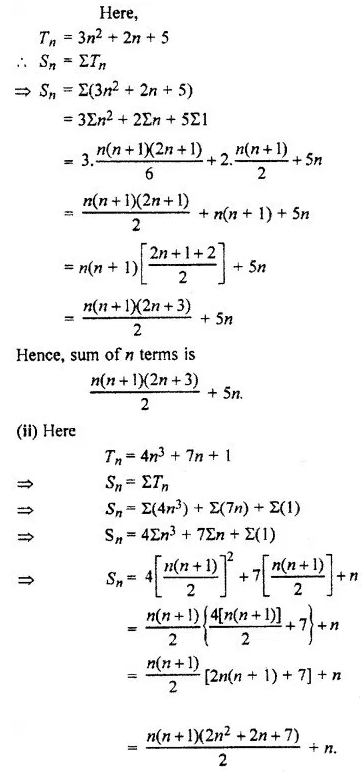Question 2: Find the sum of the terms of the series 32 + 72 + 112 + 152 + …

Solution:

32 + 72 + 112 + 152 +

nth term of the given series Tn = [3 + (n – 1)4]2

= (3 + 4n – 4)2

= (4n – 1)2

= 16n2 + 1 – 8n

∴ Sn = ∑(16n2 – 8n + 1)

⇒ Sn = 16∑n2 – 8∑M + ∑1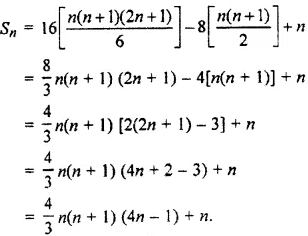Question 3: Find the nth term and sum of n terms of the series 1.3 + 3.5 + 5.7 + …

Solution:

1.3 + 3.5 + 5.7 + …

nth term of the given series Tn = (1 + 3 + 5 + … nth term)

(3 + 5 + 7 + … nth term)

= [1 + (n – 1)2 [3 +(n – 1)2]

= (1 + 2n – 2) (3 + 2n – 2)

= (2n – 1) (2n + 1)

∴ Sn = ∑ (4n2 – 1)

= 4∑n2 – ∑1

= 4 {[n (n + 1) (2n + 1)] / 6} – n

= [[2 / 3] n (n + 1) (2n + 1)] – n

= [n / 3] [2 (n + 1) (2n + 1) – 3]

= [n / 3] [4n2 – 6n + 1 – 2]

= [n / 3] [4n2 + 6n -1]

### RBSE Maths Chapter 9: Exercise 9.7 Textbook Important Questions and Solutions

Question 1: Find the term mentioned in the harmonic progression [1 / 2], [1 / 5] , [1 / 8], [1 / 11] …. 6th term.

Solution:

The A.P. corresponding to given H.P. is 2, 5, 8, 11, …

For this A.P. a = 2, d = 5 – 2 = 3

∴ T6 = a + 5d = 2 + 5 × 3

= 2 + 15

= 17

Hence, the 6th term of corresponding H.P. is = 1 / 17.

Question 2: Find the nth term of the HP [1 / 5], [2 / 9], [1 / 4], [2 / 7] ……

Solution:

The A.P. corresponding to given H.P. is 10, 9, 8, 7, …

For this A.P. a = 10, d = 9 – 10 = – 1

∴Tn = a + (n – 1)d

= 10 + (n – 1) (-1)

= 10 – n + 1

= 11 – n

Hence, the nth term of corresponding H.P. is = 2 / [11 – n].

Question 3: Find the 4 harmonic means between 1 and 1 / 16.

Solution:

Let H1, H2, H3, H4 are the 4 H.M. between 1 and 1 / 16.

So, 1, H1, H2, H3, H4 are in H.P.

The first term of corresponding A.P. is 1 and 6th term is 16.

∴ a + 5d = 16 (∵T6 = a + 5d)

⇒ 1 + 5d = 16

⇒ 5d = 15

⇒ d = 3

There will be 4 AM. between 1 and 16.

1 + d, 1 + 2d, 1 + 3d, 1 + 4d or

1 + 3, 1 + 2 (3), 1 + 3 (3), 1 + 4 (3) or

4, 7, 10, 13

So, the required HM is [1 / 4], [1 / 7], [1 / 10], [1 / 13].

Question 4: If a, b, c are in H.P., then prove that a, a – c, a – b will be in H.P.

Solution:

Let x = a, y = a – c, z = a – b are in HP.

Then by the property of H.P.

[x – y] / [y – z] = x / z or

{[a – (a – c)] / [a – c] – [a – b]} = a / [a – b]

c / [b – c] = a / [a – b] [a – b] / [b – c] = a / c

Thus, a, b, c are in H.P. which are given.

Hence, by the hypothesis i.e., a, a – c, a – b is in H.P. is true.

Question 5: If a, b and c are in HP, then prove that [1 / (b – a)] + [1 / (b – c)] + [1 / a] + [1 / c] = [2 / b].

Solution: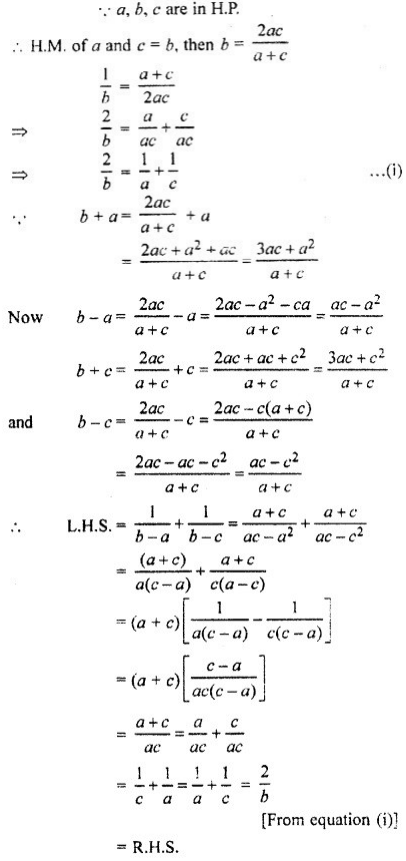### RBSE Maths Chapter 9: Exercise 9.8 Textbook Important Questions and Solutions

Question 1: A.M. of two numbers is 60 and H.M. is 18, find the numbers.

Solution:

Let the two numbers are a and b, then

[a + b] / 2 = 50

⇒ a + b = 100 and

[2ab / (a + b)] = 18

From equation (i) and (ii)

2ab / 100 = 18

⇒ ab = 900

Now (a – b)2 = (a + b)2 – 4ab

= (100)2 – [4 × 900]

= 10000 – 3600

= 6400

a – b = 80

Now, solving a + b = 100 and a – b = 80.

We get a = 90 and b = 10

Hence, the numbers are 90 and 10.

Question 2: Three numbers a, b, c are in GP. and ax = by = cz, then prove that x, z will be in H.P.

Solution:

Let ax = by = cz = k

Then a = k1/x , b = k1/y , c = k1/2 and a, b, c are in GP.

∴ b2 = ac …(i)

Put the values of a, b, c in equation (i)

k1/y = k1/x * k1/z

k2/y = k1/x+1/z which is only possible, when

2 / y = 1 / x + 1 / z

This shows that [1 / x], [1 / y], [1 / z] are in A.P.

Then x, y, z will be in H.P.

Question 3: Three numbers a, b, c are in H.P. Prove that 2a – b, b, 2c – b will be in GP.

Solution:

If a, b, c are in HP, then

[1 / b] – [1 / a] = [1 / c] – [1 / b] [2 / b] = [1 / a] + [1 / c] —- (1)

2a – b, b, 2c – b will be in GP.

If b2 = (2a – b) (2c – b)

b2 = 4ac – 2bc – 2ab + b2

4ac = 2bc + 2ab

Divide by 2abc on both sides,

4ac / 2abc = 2bc / 2abc + 2ab / 2abc

[2 / b] = [1 / a] + [1 / c] —- (2)

It is clear that from equation (i) and (ii), if a, b, c are in H.P. then 2a – b, b, 2c – b will be in G.P.

Question 4: If a, b, c are in A.P., b, c, d are in GP. and c, d, e are in H.P., then prove that a, c, e will be in H.P.

Solution:

∵ a, b, c are in A.P.

b = [a + c] /  —- (1) and

b, c, d are in GP then

c2 = bd —- (2)

Again c, d, e are in HP,

d = [2ce] / [c + e] —- (3)

Put the value of b and d from (1) and (3) in equation (2),

c2 = bd

c2 = [a + c] /  * [2ce] / [c + e]

c (c + e) = e (a + c)

c2 = ae

So, a,c and e are in GP.

Question 5: If three numbers a, b, c are in A.P. and H.P. both then prove that they will be in GP. also.

Solution:

a, b, c will be in A.P. and H.P.

b = [a + c] /  —- (1)

b = [2ac] / [a + c]

= [ac] / {[a + c] / }

= ac / b

b * b = ac

b = √ac

Hence a, b and c are in GP.